Function Repository Resource:

# HessianMatrix

Compute the Hessian matrix of a function with respect to a list of variables

Contributed by: Wolfram|Alpha Math Team
 ResourceFunction["HessianMatrix"][expr,{var1,var2,…}] computes the Hessian matrix of the expression expr with respect to the given variables.

## Examples

### Basic Examples (3)

Compute the Hessian matrix of an expression:

 In:=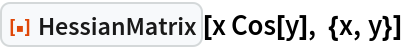Out=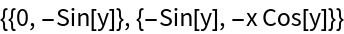Compute the Hessian matrix of another expression:

 In:=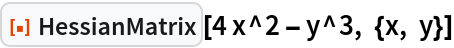Out=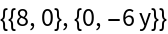Compute the Hessian matrix of another expression:

 In:=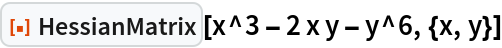Out=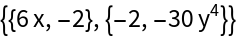Take the determinant of this result:

 In:=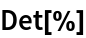Out=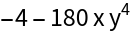Compare this to the result obtained using of ResourceFunction["HessianDeterminant"]:

 In:=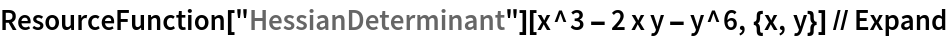Out=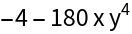## Publisher

Wolfram|Alpha Math Team

## Version History

• 4.0.0 – 23 March 2023
• 3.1.0 – 11 May 2021
• 3.0.0 – 24 January 2020
• 2.0.0 – 06 September 2019
• 1.0.0 – 05 August 2019